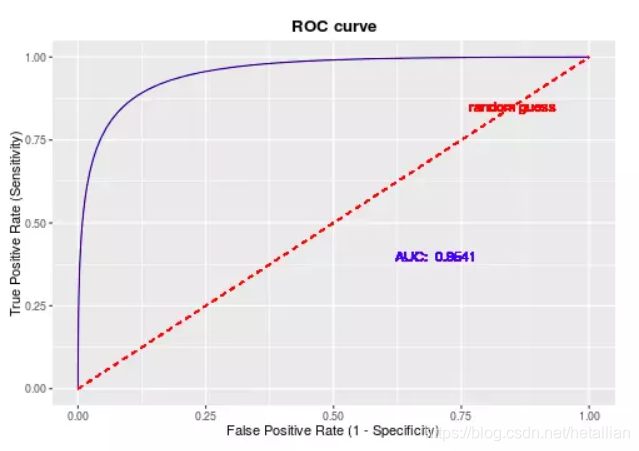# 模型常用评估指标详解- 混淆矩阵/Recall/ROC/AUC/F1/MAPE/RMSE

## 0-1分类预测的评估

### 混淆矩阵

Predicted Positive (P’=TP+FP) Predicted Negative (N’=FN+TN) TP+FP+FN+TN

• 准确率(accuracy)
• T

P

+

T

N

T

P

+

F

P

+

F

N

+

T

N

\frac{T P+T N}{TP+FP+FN+TN}

• 错误率
• F

P

+

F

N

T

P

+

F

P

+

F

N

+

T

N

\frac{FP+FN}{TP+FP+FN+TN}

• 敏感度(sensitivity)、真正例率、召回率(recall)
• T

P

T

P

+

F

N

\frac{TP}{TP+FN}

• 特效性(specificity)、真负例率
• T

N

F

P

+

T

N

\frac{TN}{FP+TN}

• 精度(precision)
• T

P

T

P

+

F

P

\frac{TP}{TP+FP}

• F

1

F_1

、F分数（精度和召回率的调和均值）

• 2

×

precision

×

recall

precision

+

r

e

c

a

l

l

=

F

1

=

2

T

P

2

T

P

+

F

P

+

F

N

\frac{2 \times \text {precision} \times \text {recall}}{\text {precision}+r e c a l l} = \mathrm{F} 1=\frac{2 \mathrm{TP}}{2 \mathrm{TP}+\mathrm{FP}+\mathrm{FN}}

• 实际来自于：

2

F

1

=

1

P

+

1

R

\frac{2}{\mathrm{F} 1}=\frac{1}{\mathrm{P}}+\frac{1}{\mathrm{R}}

### ROC曲线

• 横坐标：假正率（FPR,即 1-specificity，1-真负例率）
• FPR = FP /（FP + TN）
• 纵坐标：真正例率（TPR, 即 recall
• TPR = TP /（TP + FN）ROC曲线优势就是，当正负样本的分布发生变化时，其形状能够基本保持不变，因此其面积AUC值，可以说是极度适用于不平衡样本（例如以上的银行逾期数据，正负比极度不均衡）的建模评估了。AUC越大，模型分类效果越好，一般来说，AUC低于0.7的模型基本上是个废柴了。（若在正负样本比1:1情况下全预测为0或者1，AUC即为0.5）

### R语言实现

library(ROCR)
get_confusion_stat <- function(pred,y_real,threshold=0.5){
# auc
tmp <- prediction(as.vector(pred),y_real)
auc <- unlist(slot(performance(tmp,'auc'),'y.values'))
# statistic
pred_new <- as.integer(pred>threshold)
tab <- table(pred_new,y_real)
if(nrow(tab)==1){
print('preds all zero !')
return(0)
}
TP <- tab[2,2]
TN <- tab[1,1]
FP <- tab[2,1]
FN <- tab[1,2]
accuracy <- round((TP+TN)/(TP+FN+FP+TN),4)
recall_sensitivity <- round(TP/(TP+FN),4)
precision <- round(TP/(TP+FP),4)
specificity <- round(TN/(TN+FP),4)
# 添加，预测的负例占比（业务解释：去除多少的样本，达到多少的recall）
neg_rate <- round((TN+FN)/(TP+TN+FP+FN),4)
re <- list('AUC' = auc,
'Confusion_Matrix'=tab,
'Statistics'=data.frame(value=c('accuracy'=accuracy,
'recall_sensitivity'=recall_sensitivity,
'precision'=precision,
'specificity'=specificity,
'neg_rate'=neg_rate)))
return(re)
}


# $AUC #  0.8406198 #$Confusion_Matrix
# y_real
# pred_new   0   1
# 0  20   8
# 1  32 131

# \$Statistics
# value
# accuracy           0.7906
# recall_sensitivity 0.9424
# precision          0.8037
# specificity        0.3846
# neg_rate           0.1466


## 连续值预测的评估

### MAPE

MAPE（mean absolute percentage error）为平均百分比误差，预测连续型数据的准确率一般指1-MAPE，例如预测未来10个月的GDP数据，准确率达到98%，即代表该模型的预测 MAPE 为2%

M

A

P

E

=

t

=

1

n

observed

t

predicted

t

observed

t

×

100

n

M A P E=\sum_{t=1}^{n}\left|\frac{\text {observed}_{t}-\text {predicted}_{t}}{\text {observed}_{t}}\right| \times \frac{100}{n}

MAPE=t=1nobservedtobservedtpredictedt×n100

### MSE/RMSE

RMSE(root mean square error)为均方根误差，相应的MSE(mean square error)即为误差的平方和，两者含义一致，指标越小则模型效果越好。

R

M

S

E

=

1

N

t

=

1

N

(

observed

t

predicted

t

)

2

R M S E=\sqrt{\frac{1}{N} \sum_{t=1}^{N}\left(\text {observed}_{t}-\text {predicted}_{t}\right)^{2}}

RMSE=N1t=1N(observedtpredictedt)2

### 拟合优度

#### R²/可决系数

R

2

=

S

S

r

e

g

S

S

t

s

s

=

1

S

S

r

s

s

S

S

t

s

s

=

1

i

(

y

i

f

i

)

2

i

(

y

i

y

ˉ

)

2

R^{2} = \frac{S S_{\mathrm{reg}}}{S S_{\mathrm{tss}}}=1-\frac{S S_{\mathrm{rss}}}{S S_{\mathrm{tss}}} = 1- \frac{\sum_{i}\left(y_{i}-f_{i}\right)^{2}}{\sum_{i}\left(y_{i}-\bar{y}\right)^{2}}

R

2

R^2

R2就是预测值和实际值相关系数的平方，即（注意，一定是线性回归模型才行）：

R

2

=

c

o

r

(

y

r

e

a

l

,

y

f

i

t

)

2

R^{2}=cor(y_{real},y_{fit})^{2}

R2=cor(yreal,yfit)2

R

a

d

j

u

s

t

e

d

2

=

1

S

S

r

s

s

/

(

n

p

1

)

)

S

S

t

s

s

/

(

n

1

)

### R语言实现

# MAPE
get_mape <- function(fit,y){
# 实际值为0的，直接不纳入计算
re <- round(mean(ifelse(y==0,NA,abs(y-fit)/y),na.rm=T)*100,1)
return(re)
}

# R方，可决系数，coefficient of determination
get_rsq <- function(preds,actual,p=1){
rss <- sum((actual - preds) ^ 2)
tss <- sum((actual - mean(actual)) ^ 2)
rsq <- round(1 - rss/tss,3)
n <- length(preds)
# 调整的R方 1-(1-rsq)*(n-1)/(n-1-1) 也一样